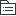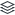# 第九章 资本结构

CAP财务管理第九章资本结构。

72
|
14

## 相关模板推荐

•## 资本结构 —— 作品大纲

•资本结构理论
• 1、MM理论
• 无税MM理论
• 命题 1
• 1、资本结构与企业价值无关，企业的加权平均成本与其资本结构无关
• 2、有负债的企业价值=无负债的企业价值
• 3、有负债企业的加权平均资本成本=经营风险等级相同的无负债企业的权益资本成本
• 命题2
• 1、有负债的企业权益资本成本随着财务杠杆的提高而增加
• 2、有负债企业的权益成本=无负债企业的权益成本+风险溢价
• 3、风险溢价与以市值计算的财务杠杆（债务/权益）成正比例
• 有税MM理论
• 命题1
• 1、企业负债比例提高，企业价值也随之提高，在理论上全部融资来源为负债时价值最大
• 2、有负债企业的价值=具有相同风险等级的无负债企业的价值+债务利息抵税收益的现值
• 命题2
• 1、有负债企业的权益资本成本随着财务杠杆的提高而增加
• 2、有负债企业的权益资本成本=相同风险等级的无负债企业的权益资本成本+以市值计算的债务与权益比例成比例的风险报酬(风险溢价=（无负债企业的权益成本-税前债务资本成本）*（1-T）*D/E)
• 3、风险报酬取决于企业的债务比例以及所得税税率
• 2、权衡理论
• VL=VU+PV(利息抵税)-PV（财务困境成本）
• 3、代理成本
• 过度投资
• 投资不足
• 4、优序融资理论
• 1、内源融资
• 2、债务融资
• 3、股权融资
•资本结构决策分析
• 1、资本成本比较法
• 加权平均资本成本最低，企业价值最大
• 2、每股收益无差别点法
• 每股收益EPS=[（EBIT-I）*(1-T)-PD]/N
• 3、企业价值比较法
• 企业的市场价值V=股票的市场价值S+长期债务的价值B+优先股的价值P
• 股票市场价值S=[（EBIT-I）*(1-T)-PD]/rs
• rs为权益资本成本
• rs=无风险利率+贝塔值*市场风险溢价
• 加权平均资本成本rWACC=rd*(1-T)*B/V+rs*S/V
•杠杆系数的衡量
• 经营风险
• 经营杠杆DOL=息税前利润变化的百分比/营业收入变化的百分比
• DOL=（变化的EBIT/EBIT ）/（营业收入S的变化/营业收入S）
• 销售量为Q时的经营杠杆DOLQ=Q*(P-V)/(Q*(P-V)-F)
• 营业收入为S时的经营杠杆DPLS=（S-VC）/(S-VC-F)=(EBIT+F)/EBIT
• 增加营业收入、降低单位变动成本、降低固定成本比重等降低经营风险
• 财务风险
• 财务杠杆DFL=每股收益的变化的百分比/息税前利润变化的百分比
• DFL=(EPS的变化/EPS)(EBIT的变化值/EBIT)
• DFL=EBIT/(EBIT-I-PD/(1-T))
• DFL=(Q*(P-V)-F)/(Q*(P-V)-F-I-PD/(1-T))
• 联合杠杆
• DTL=DOL*DFL=（EPS的变化值/EPS）/（营业收入S的变化值/营业收入S)
• DTL=Q*(P-V)/(Q*(P-V)-F-I-PD/(1-T))
• DTL=(EBIT+F)/(EBIT-I-PD/(1-T))